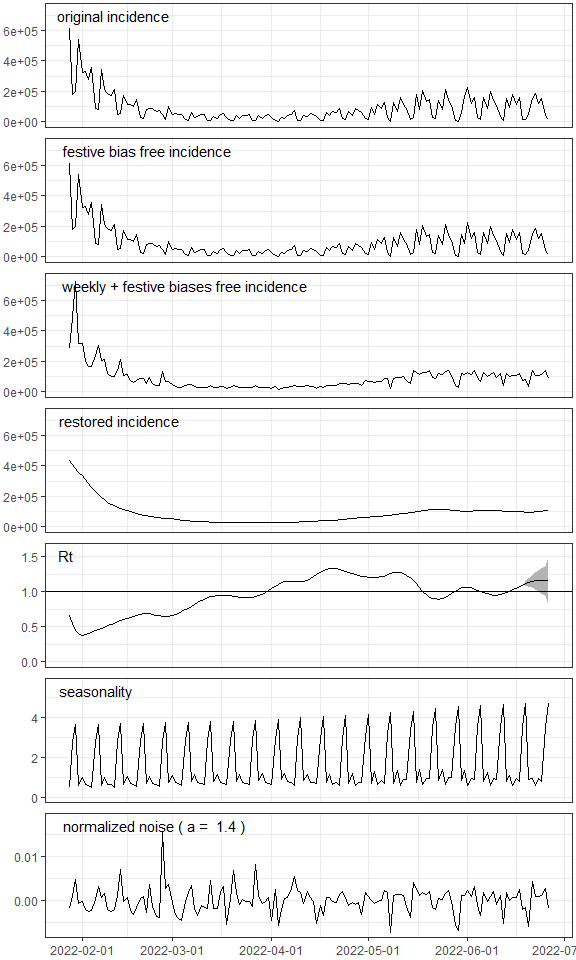# EpiInvert

## Overview

Using the daily incidence curve and a collection of festive or anomalous days EpiInvert estimates a time-varying reproduction number and a restored incidence curve. EpiInvert is founded on a variational formulation inverting a renewal equation which includes the following features:

• Correction of the administrative weekly bias using 7-day quasi-periodic multiplicative correction factors.
• Correction of the bias introduced by the festive or anomalous days where we know “a priori” that the registered number of cases is biased.
• Management of weekly aggregated incidence data.
• Incidence modelization (see equation (5) below).

EpiInvert inverts the renewal equation :

$$(1) \ \ \ \ i_t=\sum_k i_{t-k}R_{t-k}\Phi_k$$

through a variational model as described in PNAS, 2021 and Biology, 2022. See
Rt comparison for a comparison with other methods which compute the reproduction number.

A festive or anomalous day is any day where we know “a priori” that the registered number of cases is biased. Typically, in those days, one observes a sharp decrease in the number of registered incidence that is compensated by increased incidence numbers in the next few days. This bias is corrected by redistributing the number of cases in the festive day and the next 2 days.

On top of the festive day bias, there is a strong administrative weekly bias introduced by the way the countries registered the new cases each day of the week. This weekly bias is corrected using 7-day quasi-periodic multiplicative correction factors. We use the following notation :

$$(2) \ \ \ \ i^f_t \ \ \text{is the festive day bias free incidence}$$

$$(3) \ \ \ \ q_t \ \ \text{ is the 7-day quasi-periodic multiplicative correction factor}$$

$$(4) \ \ \ \ i^b_t=i^f_tq_t \ \ \text{is the festive + weekly biases free incidence}$$

Once the festive day and weekly biases are corrected in the incidence curve the difference between the incidence curve and its expected value using the renewal equation is modeled by

$$(5) \ \ \ \ i^b_{t}=i^r_t+\varepsilon_{t}(i_{t}^r)^a$$

where

$$(6) \ \ \ \ i^r_t=\sum_k i^b_{t-k}R_{t-k}\Phi_k.$$

In a nutshell, the proposed variational model is based on estimating all the variables involved in order to minimize the difference between the incidence and its expected value using the renewal equation.

The power a in the equation (5) is computed experimentally by linear regression (in t) applied to

$$(7) \ \ \ \ (log(|i^b_{t}-i^r_t|),log(i^r_t)).$$

The normalized error of the model is given by

$$(8) \ \ \ \ \epsilon_t=\frac{i^b_t-i^r_t}{(i^r_t)^a}.$$

In Biology, 2022, it is shown experimentally that this normalized error is well approximated by an exponential distributed white noise.

To model the serial interval, EpiInvert allows a shifted log-normal parametric formulation. The shift can be negative, reflecting the fact that secondary cases may present symptoms earlier than the primary case. The user can also provide a non-parametric serial interval given by a numeric vector.

## Package installation

You can install the development version of EpiInvert from GitHub with:

 install.packages("devtools")
devtools::install_github("lalvarezmat/EpiInvert")

## Examples

We attach some required packages

library(EpiInvert)
library(ggplot2)
library(dplyr)
library(grid)

Loading stored data on COVID-19 daily incidence up to 2022-05-05 for France, Germany, the USA and the UK:

data(incidence)
tail(incidence)
#>           date   FRA    DEU    USA    UK
#> 828 2022-04-30 49482  11718  23349     0
#> 829 2022-05-01 36726   4032  16153     0
#> 830 2022-05-02  8737 113522  81644    32
#> 831 2022-05-03 67017 106631  61743 35518
#> 832 2022-05-04 47925  96167 114308 16924
#> 833 2022-05-05 44225  85073  72158 12460

Loading some festive days for the same countries:

data(festives)
#>          USA        DEU        FRA         UK
#> 1 2020-01-01 2020-01-01 2020-01-01 2020-01-01
#> 2 2020-01-20 2020-04-10 2020-04-10 2020-04-10
#> 3 2020-02-17 2020-04-13 2020-04-13 2020-04-13
#> 4 2020-05-25 2020-05-01 2020-05-01 2020-05-08
#> 5 2020-06-21 2020-05-21 2020-05-08 2020-05-25
#> 6 2020-07-03 2020-06-01 2020-05-21 2020-06-21

### Example 1

We show the execution of EpiInvert using Germany data. The first parameter is a numerical vector with the daily incidence, the second parameter is the date of the last incidence value and the third parameter is a character vector with the festive days (this parameter is not mandatory)

res <- EpiInvert(incidence$DEU,"2022-05-05",festives$DEU)

Plotting the results:

EpiInvert_plot(res)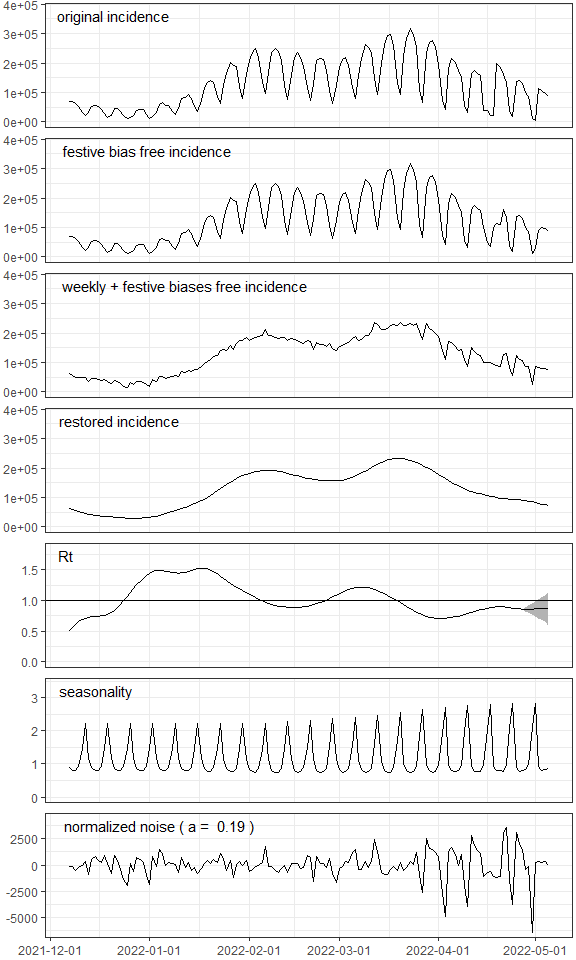EpiInvert return a list with the following elements:

• i_original : the original daily incidence curve. Notice that EpiInvert does not allow missing values. On days when a country does not report data, a zero must be registered as the value associated with the incidence of that day.

• i_festive : the festive days bias free incidence (see equation (2)).

• i_bias_free : the festive days and weekly biases free incidence (see equation (4)).

• i_restored : the restored incidence (see equation (6)).

• Rt : time varying reproduction number.

• Rt_CI95 : to estimate Rt on each day t, EpiInvert uses the past days (t’<=t) and the future days (t’>t) when available. Therefore, the EpiInvert estimate of Rt varies when more days are available. Rt_CI95 represents the radius of an empiric 95% confidence interval of the expected variation of Rt as a function of the number of days after t available (see the plot of Rt above).

• seasonality : the 7-day quasi-periodic multiplicative correction factors (see equations (3)-(4)).

• dates : the date associated with each incidence value.

• festive : a Boolean vector to indicate the days considered as festive.

• epsilon : the normalized error given by equation (8).

• power_a : the power a in equation (8). Note that this value strongly depends on the size of the incidence curve used by the EpiInvert estimation. As we shall see later, this size is an EpiInvert parameter. The estimated value of a only has sense in the case of a large incidence sequence.

• si_distr : numeric vector with the serial interval distribution used, it can be computed from the shifted parametric log-normal or it can be uploaded by the user

• shift_si_distr : shift of the serial interval used.

### Example 2

EpiInvert execution for France using 365 days in the past. If you are not constrained by the computational cost of the algorithm, you can choose a large value of this parameter (for instance 9999), to ensure that EpiInvert will use the whole available sequence in the estimation.

res <- EpiInvert(incidence$FRA,"2022-05-05",festives$FRA,
select_params(list(max_time_interval = 365)))

Plot of the incidence between “2021-12-15” and “2022-01-15”. Observe that the festive days bias correction only modifies the original incidence in the festive days and the following 2 days.

 EpiInvert_plot(res,"incid","2021-12-15","2022-01-15")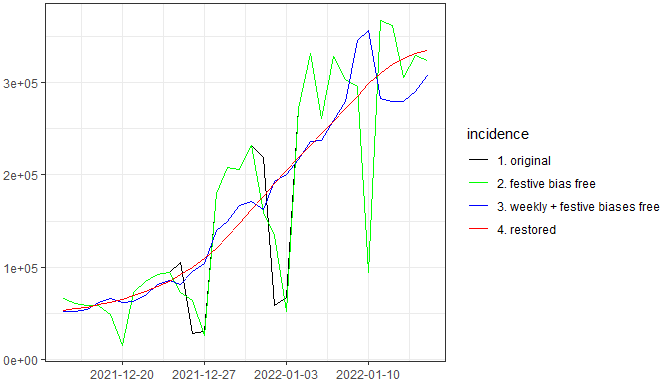### Example 3

EpiInvert execution for UK using a non-parametric serial interval shifted -2 days

load data of a serial interval

data(si_distr_data)
#>  3.285609e-06 3.401902e-04 3.904441e-03 1.543537e-02 3.466818e-02
#>  5.608451e-02
res <- EpiInvert(incidence$UK,"2022-05-05",festives$UK,
select_params(list(si_distr = si_distr_data,
shift_si_distr=-2)))

Plot of the serial interval used (including the shift)

 EpiInvert_plot(res,"SI")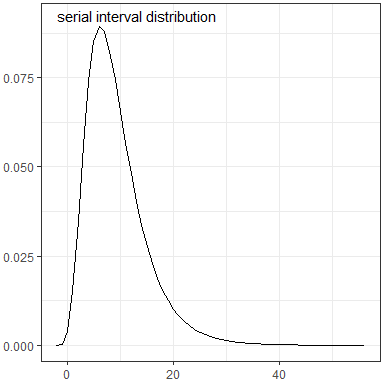### Example 4

EpiInvert execution for the USA changing the default values of the parametric serial interval (using a shifted log-normal)

res <- EpiInvert(incidence$USA,"2022-05-05",festives$USA,
select_params(list(mean_si = 11,sd_si=6,shift_si=-1)))

Plot of the reproduction number Rt including an empiric 95% confidence interval of the variation of EpiInvert Rt estimation as a function of the number of future days available.

 EpiInvert_plot(res,"R")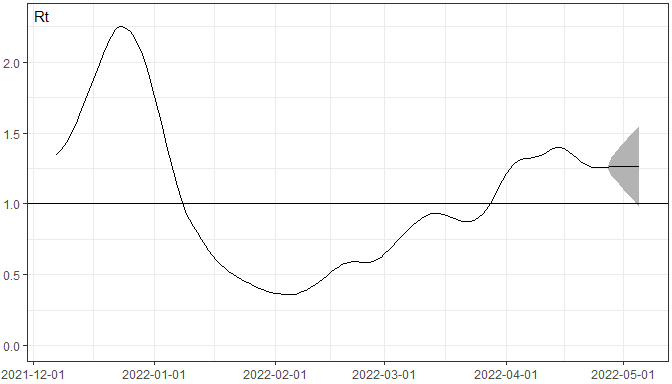### Example 5

EpiInvert execution using the weekly aggregated incidence for France. First we upload the weekly aggregated incidence values:

data(incidence_weekly_aggregated)
tail(incidence_weekly_aggregated)
#>           date    FRA     DEU    USA     UK
#> 114 2022-03-31 978532 1464011 215628 536944
#> 115 2022-04-07 934420 1084012 191636 362326
#> 116 2022-04-14 898134  898260 277703 241487
#> 117 2022-04-21 628620  666943 284320 163087
#> 118 2022-04-28 466867  704515 398596 117277
#> 119 2022-05-05 307031  504441 429406  77410

We run EpiInvert for the weekly aggregated incidence :

res2 <- EpiInvert(incidence_weekly_aggregated$FRA,"2022-05-05",festives$FRA,
select_params(list(incidence_weekly_aggregated = TRUE)))

For comparison purposes we run EpiInvert for the daily incidence

res <- EpiInvert(incidence$FRA,"2022-05-05",festives$FRA)

We create a dataframe to plot some comparative results

df <- data.frame(date = c(as.Date(res$dates),as.Date(res2$dates)),
i_restored = c(res$i_restored,res2$i_restored),
i_original = c(res$i_original,res2$i_original),
Rt = c(res$Rt,res2$Rt),
legend = c(rep("daily data", length(res$i_restored)),rep("weekly aggregated", length(res2$i_restored))))

Next, we present the plot of the original incidence curves used by EpiInvert. In the case of the of the weekly aggregated incidence we initialize the original curve assigning each day of the week 1/7 of the weekly aggregated value:

 ggplot(df,aes(date, i_original, col = legend))+ geom_line(size = 0.7)+theme(legend.position="bottom")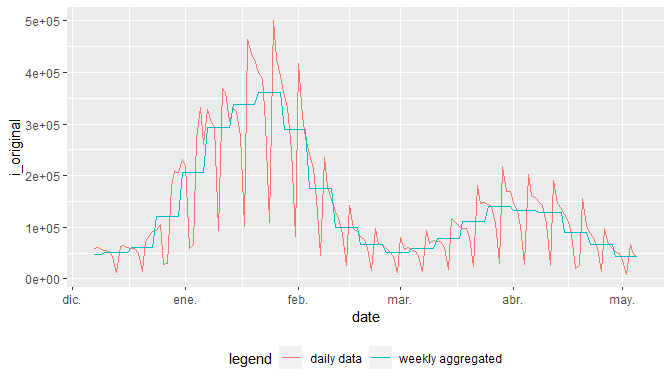Next, we present the plot of the restored incidence curves for the daily and the weekly aggregated incidence. We observe that due to the regularization effect of the variational model both curves are quite similar.

ggplot(df,aes(date, i_restored, col = legend))+ geom_line(size = 0.7)+theme(legend.position="bottom")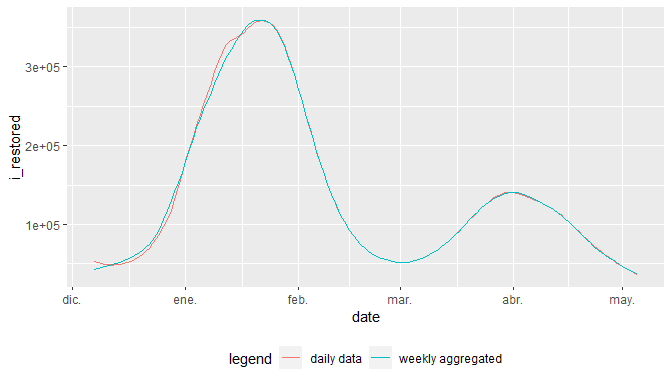Next, we present the plot of the reproduction number Rt for the daily and the weekly aggregated incidence. Again, we observe that due to the regularization effect of the variational model both curves are quite similar.

 ggplot(df,aes(date, Rt, col = legend))+ geom_line(size = 0.7)+theme(legend.position="bottom")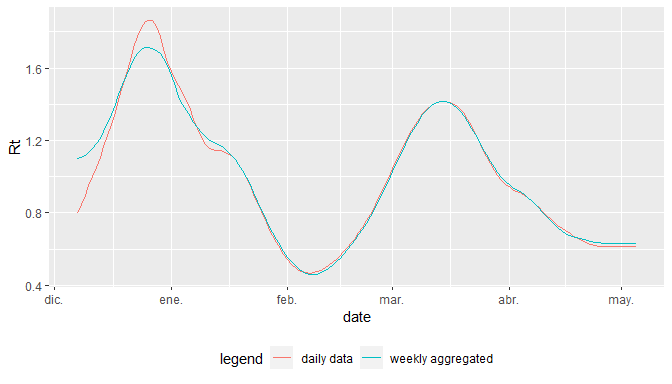### Updated version of the incidence curves

To load an updated version of the incidence file we use in these examples you can execute:

incidence <- read.csv(url("https://www.ctim.es/covid19/incidence.csv"))
tail(incidence)
#>           date   FRA    DEU    USA    UK
#> 882 2022-06-21     0 119232 155151 16430
#> 883 2022-06-22 11566 119360 184074 33137
#> 884 2022-06-23 95217 108190 121315 18269
#> 885 2022-06-24 77967  89336 152095 16473
#> 886 2022-06-25 79852      0  39372     0
#> 887 2022-06-26 79262    799  18551     0

you can introduce in the EpiInvert call the last date of the incidence using the dates included in the incidence file:

res <- EpiInvert(incidence$USA,incidence$date[length(incidence$date)],festives$USA)
 EpiInvert_plot(res)